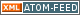www.LinuxHowtos.org howtos, tips&tricks and tutorials for linuxfrom small one page howto to huge articles all in one place

search text in:

Poll
Which screen resolution do you use?

poll results

using iotop to find disk usage hogs

using iotop to find disk usage hogs

words:

887

views:

144457

userrating:

average rating: 1.8 (88 votes) (1=very good 6=terrible)

May 25th. 2007:
Words

486

Views

243560

Workaround and fixes for the current Core Dump Handling vulnerability affected kernels

Workaround and fixes for the current Core Dump Handling vulnerability affected kernels

words:

161

views:

126464

userrating:

average rating: 1.3 (28 votes) (1=very good 6=terrible)

April, 26th. 2006:

You are here: manpages

Section: OpenSSL (3)
Updated: 2017-05-25

## NAME

BN_add, BN_sub, BN_mul, BN_sqr, BN_div, BN_mod, BN_nnmod, BN_mod_add, BN_mod_sub, BN_mod_mul, BN_mod_sqr, BN_exp, BN_mod_exp, BN_gcd - arithmetic operations on BIGNUMs

## SYNOPSIS

``` #include <openssl/bn.h>

int BN_add(BIGNUM *r, const BIGNUM *a, const BIGNUM *b);

int BN_sub(BIGNUM *r, const BIGNUM *a, const BIGNUM *b);

int BN_mul(BIGNUM *r, BIGNUM *a, BIGNUM *b, BN_CTX *ctx);

int BN_sqr(BIGNUM *r, BIGNUM *a, BN_CTX *ctx);

int BN_div(BIGNUM *dv, BIGNUM *rem, const BIGNUM *a, const BIGNUM *d,
BN_CTX *ctx);

int BN_mod(BIGNUM *rem, const BIGNUM *a, const BIGNUM *m, BN_CTX *ctx);

int BN_nnmod(BIGNUM *r, const BIGNUM *a, const BIGNUM *m, BN_CTX *ctx);

int BN_mod_add(BIGNUM *r, BIGNUM *a, BIGNUM *b, const BIGNUM *m,
BN_CTX *ctx);

int BN_mod_sub(BIGNUM *r, BIGNUM *a, BIGNUM *b, const BIGNUM *m,
BN_CTX *ctx);

int BN_mod_mul(BIGNUM *r, BIGNUM *a, BIGNUM *b, const BIGNUM *m,
BN_CTX *ctx);

int BN_mod_sqr(BIGNUM *r, BIGNUM *a, const BIGNUM *m, BN_CTX *ctx);

int BN_exp(BIGNUM *r, BIGNUM *a, BIGNUM *p, BN_CTX *ctx);

int BN_mod_exp(BIGNUM *r, BIGNUM *a, const BIGNUM *p,
const BIGNUM *m, BN_CTX *ctx);

int BN_gcd(BIGNUM *r, BIGNUM *a, BIGNUM *b, BN_CTX *ctx);

```

## DESCRIPTION

BN_add() adds a and b and places the result in r ("r=a+b"). r may be the same BIGNUM as a or b.

BN_sub() subtracts b from a and places the result in r ("r=a-b").

BN_mul() multiplies a and b and places the result in r ("r=a*b"). r may be the same BIGNUM as a or b. For multiplication by powers of 2, use BN_lshift(3).

BN_sqr() takes the square of a and places the result in r ("r=a^2"). r and a may be the same BIGNUM. This function is faster than BN_mul(r,a,a).

BN_div() divides a by d and places the result in dv and the remainder in rem ("dv=a/d, rem=a%d"). Either of dv and rem may be NULL, in which case the respective value is not returned. The result is rounded towards zero; thus if a is negative, the remainder will be zero or negative. For division by powers of 2, use BN_rshift(3).

BN_mod() corresponds to BN_div() with dv set to NULL.

BN_nnmod() reduces a modulo m and places the non-negative remainder in r.

BN_mod_add() adds a to b modulo m and places the non-negative result in r.

BN_mod_sub() subtracts b from a modulo m and places the non-negative result in r.

BN_mod_mul() multiplies a by b and finds the non-negative remainder respective to modulus m ("r=(a*b) mod m"). r may be the same BIGNUM as a or b. For more efficient algorithms for repeated computations using the same modulus, see BN_mod_mul_montgomery(3) and BN_mod_mul_reciprocal(3).

BN_mod_sqr() takes the square of a modulo m and places the result in r.

BN_exp() raises a to the p-th power and places the result in r ("r=a^p"). This function is faster than repeated applications of BN_mul().

BN_mod_exp() computes a to the p-th power modulo m ("r=a^p % m"). This function uses less time and space than BN_exp().

BN_gcd() computes the greatest common divisor of a and b and places the result in r. r may be the same BIGNUM as a or b.

For all functions, ctx is a previously allocated BN_CTX used for temporary variables; see BN_CTX_new(3).

Unless noted otherwise, the result BIGNUM must be different from the arguments.

## RETURN VALUES

For all functions, 1 is returned for success, 0 on error. The return value should always be checked (e.g., "if (!BN_add(r,a,b)) goto err;"). The error codes can be obtained by ERR_get_error(3).

## HISTORY

BN_add(), BN_sub(), BN_sqr(), BN_div(), BN_mod(), BN_mod_mul(), BN_mod_exp() and BN_gcd() are available in all versions of SSLeay and OpenSSL. The ctx argument to BN_mul() was added in SSLeay 0.9.1b. BN_exp() appeared in SSLeay 0.9.0. BN_nnmod(), BN_mod_add(), BN_mod_sub(), and BN_mod_sqr() were added in OpenSSL 0.9.7.

## Index

NAME
SYNOPSIS
DESCRIPTION
RETURN VALUES
HISTORY||- Powered by- Running on-
Copyright 2004-2020 Sascha Nitsch Unternehmensberatung GmbH::- Level Triple-A Conformance to Web Content Accessibility Guidelines 1.0 -
- Copyright and legal notices -